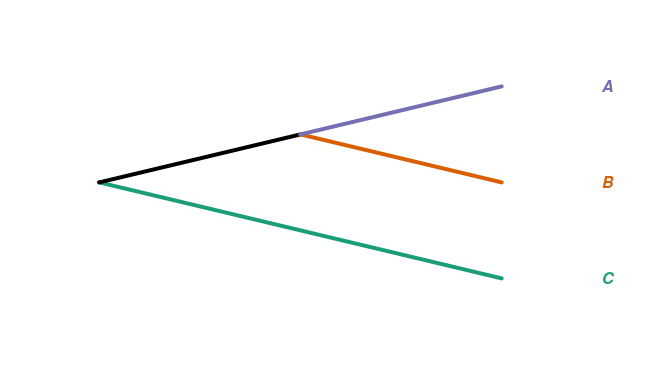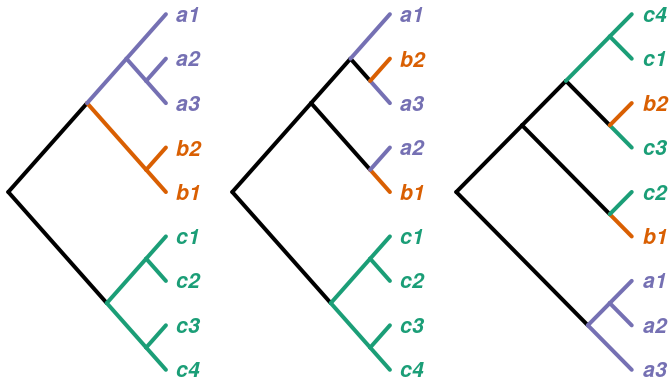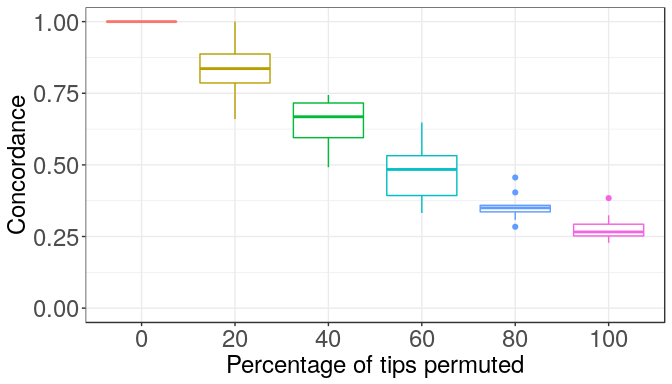Comparing trees by tip label categories

2019-04-07

We introduce distance measures between trees with ‘related’ tip labels in a recent bioRxiv preprint, Comparing phylogenetic trees according to tip label categories. Here we provide an overview of the measures and present some simple examples.

# load treespace and packages for plotting:
library(treespace)
library(RColorBrewer)
library(ggplot2)
library(reshape2)
# set colour scheme
pal <- brewer.pal(3,"Dark2")

Suppose you have a tree with taxa labels which correspond to some broad “categories” and you want to compare it to other tree(s) whose taxa also correspond to those categories. If the tip labels of the trees do not match exactly then the other metrics supplied in treespace cannot be applied. The function relatedTreeDist is a version of treeDist (the Kendall Colijn metric) which compares tip labels at their category levels, and treeConcordance is a measure of how “concordant” a tree is to a reference tree linking the categories, as we shall explain below.

Some examples of instances where we may wish to compare trees with such labels:

Categories Individuals
Bacterial sub-types e.g. serogroups Bacterial isolates
Species Orthologous genes
Host Deep sequencing reads of pathogen
Protein families Proteins
Population groups Individual organisms
Disjoint features or phenotypes Individual organisms
Broad taxonomy Individual organisms

Concordance

The concordance measure takes a reference tree R whose tips are a set of categories (with no repeats), and a comparable tree T whose tips are individuals from those categories. The measure counts the proportion of tip pairs whose MRCA in T appears at the same place as the MRCA of their categories in R. It takes a value in (0,1]. Full concordance, where the collapsed version of T is identical to R, gives a value of 1.

For example,

plot(catTree, tip.color=pal,
edge.width = 4, type="cladogram",
label.offset= 0.5, font=4,
edge.color=c(pal[],"black",pal[],pal[]))layout(matrix(1:3,1,3))
plot(indTree1, tip.color=c(rep(pal[],4),rep(pal[],2),rep(pal[],3)),
edge.width = 4, type="cladogram", no.margin=TRUE,
label.offset= 0.5, font=4, cex=2,
edge.color=c("black",rep(pal[],6),"black",rep(pal[],3),rep(pal[],5)))
plot(indTree2, tip.color=c(rep(pal[],4),pal[],rep(pal[],2),pal[],pal[]),
edge.width = 4, type="cladogram", no.margin=TRUE,
label.offset= 0.5, font=4, cex=2,
edge.color=c("black",rep(pal[],6),rep("black",2),pal[],pal[],
rep("black",2),pal[],pal[],pal[]))
plot(indTree3, tip.color=c(rep(pal[],3),pal[],rep(pal[],2),pal[],rep(pal[],2)),
edge.width = 4, type="cladogram", no.margin=TRUE,
label.offset= 0.5, font=4, cex=2,
edge.color=c("black",rep(pal[],4),rep("black",2),pal[],pal[],
rep("black",2),pal[],pal[],rep(pal[],3)))The first tree has monophyly per category, and the relative positions of those categories are identical to the reference tree. Correspondingly, the concordance is 1:

df <- cbind(c(rep("A",3),rep("B",2),rep("C",4)),sort(indTree1$tip.label)) treeConcordance(catTree,indTree1,df) ##  1 The second tree is fairly similar to the reference, with category C monophyletic and basal to the rest. However, the paraphyly of categories A and B gives a concordance less than 1: treeConcordance(catTree,indTree2,df) ##  0.8846 Finally, the third tree has much less in common with the reference and accordingly has lower concordance: treeConcordance(catTree,indTree3,df) ##  0.4615 To give a broader feel for how the concordance measure behaves, we now perform an experiment where we create random trees and compare them to a reference. We permute the tips in the individuals trees by a given percent, and watch the concordance decrease as the permutations increase and the category-level relationships weaken. In the paper we report our findings for n=10 and reps=100, that is, the reference tree has 10 tips, individuals trees have n*n=100 tips, and we performed 100 repetitions for each level of permutation. To keep the calculations quick we only use n=5 and reps=10 here. n <- 5 reps <- 10 reftree <- rtree(n, tip.label=letters[1:n]) indTrees <- lapply(rep(seq(0,100,20),reps), function(x) simulateIndTree(reftree,itips=n,permuteTips=TRUE,tipPercent=x)) df <- cbind(sort(rep(letters[1:n],n)),sort(indTrees[]$tip.label))

concordances <- sapply(indTrees, function(x) treeConcordance(reftree,x,df))

resultsTab <- as.data.frame(cbind(rep(seq(0,100,20),reps),concordances))
colnames(resultsTab) <- c("Percentage","Concordance")
resultsTab[,1] <- factor(resultsTab[,1], levels=seq(0,100,20))
plot <- ggplot(resultsTab, aes(x=Percentage, y=Concordance))

plot + geom_boxplot(aes(colour=Percentage)) + theme_bw() + guides(colour=FALSE) +
xlab("Percentage of tips permuted") + ylim(c(0,1)) +
theme(axis.text = element_text(size=18),
axis.title = element_text(size=18))